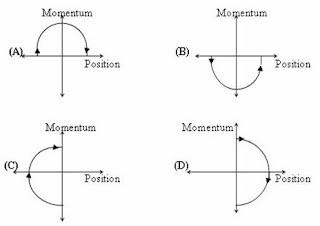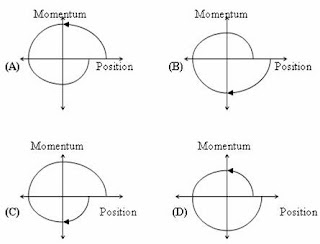## Friday, April 15, 2011

### IIT-JEE 2011 – Paragraph Type Multiple Choice (Single Answer) Questions Involving Phase Space Diagrams

“The earth provides enough to satisfy every man’s needs, but not every man’s greed.”

Mahatma Gandhi

The practice of asking two or three multiple choice questions (often single answer type) based on a given paragraph, is resorted to in many entrance examinations. The following three questions[(1), (2) and (3)] which appeared in IIT-JEE 2011 question paper are relatively simple:

Paragraph for questions (1), (2) and (3)

Phase space diagrams are useful tools in analyzing all kinds of dynamical problems. They are especially useful in studying the changes in motion as initial position and momentum are changed. Here we consider some simple dynamical systems in one–dimension. For such system, phase space is a plane is which position is plotted along horizontal axis and momentum is plotted along vertical axis. The phase space diagram is x (t) vs p(t) curve in this plane. The arrow on the curve indicates the time flow. For example, the phase space diagram for a particle moving with constant velocity is a straight line as shown in the figure. We use the sign convention in which position or momentum upwards (or to right) is positive and downwards (or to left) is negative.

(1) The phase space diagram for a ball thrown vertically up from the ground isThe arrow on the diagram shows the flow of time. Initially the ball is on the ground and its velocity and momentum have maximum positive values (since it moves upwards). At the highest point of its trajectory the displacement of the ball has maximum positive value but its momentum is zero. Finally when the ball just hits the ground, its displacement is zero but its momentum has maximum negative value(since it is moving downwards).

The correct option is obviously (D).(2) The phase space diagram for simple harmonic motion is a circle centered at the origin. In the figure, the two circles represent the same oscillator but for different initial conditions, and E1 and E2 are the total mechanical energies respectively. Then

(A) E1 = √2 E2

(B) E1 = 2 E2

(C) E1 = 4 E2

(D) E1 = 16 E2

When the total energy of the simple harmonic motion (SHM) is E1, the amplitude is 2a and when the total energy is E2, the amplitude is a, as is evident from the phase space diagrams. The total energy E of a particle of mass m in simple harmonic motion of amplitude A and angular frequency ω is given by

E = ½ 2A2

So when the amplitude is doubled, the total energy is quadrupled.

Thus E1 = 4 E2 as given in option (C).

[The phase space diagram given in the above question represents an undamped simple harmonic motion since respective amplitudes appropriate to the initial conditions remain constant. If the simple harmonic motion is a damped one, the curve will be a spiral, proceeding inwards].(3) Consider the spring-mass system, with the mass submerged in water, as shown in the figure. The phase space diagram for one cycle of this system isThe correct option is (B) on account of the following reasons:

(i) The amplitude of oscillations must decrease with time [which is not the case in diagrams (C) and (D)] as the viscous forces in water damps the motion of the system.

(ii) When the displacement is maximum positive value (corresponding to maximum positive position co-ordinate), the momentum is zero and is going to increase in magnitude in the negative direction [unlike in the case of diagram (A)].

1.2.